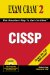# Mastering the Inner Game

### Answers to Exam Prep Questions

 A1: Answer: B. A cipher-text attack requires the attacker to obtain several encrypted messages that have been encrypted using the same encryption algorithm. Answer A is incorrect because a known plain-text attack requires the attacker to have the plain text and cipher text of one or more messages. Answer C is incorrect because there would be no reason to perform an attack if the information was already in clear text. Answer D is incorrect because a reply attack occurs when the attacker can intercept session keys and reuse them at a later date. A2: Answer: B. Cryptanalysis is the act of obtaining plain text from cipher text without a key. Cryptanalysis is the study of breaking encryption systems. Although it can mean obtaining the plain text from the cipher text without a key, it can also mean that the cryptosystem was cracked because someone found a weakness in the cryptosytem's implementation. Such was the case with wired equivalent privacy (WEP). Answer A is incorrect because although the cryptanalyst can use frequency analysis to aid in cracking, it is not a valid answer. Answer C is incorrect because encryption is the act of unencrypting data. Answer D is incorrect because cracking is a term used to describe criminal hackers and the art of illegally accessing source code. A3: Answer: D. A reply attack occurs when the attacker can intercept session keys and reuse them at a later date. Answer A is incorrect because a known plain-text attack requires the attacker to have the plain text and cipher text of one or more messages. Answer B is incorrect because a cipher-text attack requires the attacker to obtain several encrypted messages that have been encrypted using the same encryption algorithm. Answer C is incorrect because a man-in-the middle attack is carried out when the attacker places himself between two users. A4: Answer: C. Key management is a primary disadvantage of symmetric encryption. Answers A, B, and D are incorrect because encryption speed, key size, and key strength are not disadvantages of symmetric encryption. A5: Answer: D. RSA is an asymmetric algorithm. Answers A, B, and C are incorrect because DES, RC5, and AES are examples of symmetric algorithms. A6: Answer: B. Electronic Code Book is susceptible to known plain-text attacks because the same clear text always produces the same cipher text. Answers A, C, and D are incorrect. Because CBC, CFB, and OFB all use some form of feedback, they do not suffer from this deficiency and are considered more secure. A7: Answer: A. Each 64-bit plain-text block is separated into two 32-bit blocks and then processed by the 56-bit key. Total key size is 64 bits, but 8 bits are used for parity, thereby making 64, 96, and 128 bits incorrect. A8: Answer: C. DES-EEE2 performs the first and third encryption passes using the same key. Answers A, B, and D are incorrect: DES-EEE3 uses three different keys for encryption. HAVAL is used for hashing; it is not used by DES. DES-X is a variant of DES, with only a 56-bit key size. A9: Answer: C. Diffie-Hellman is used for key distribution, not encryption or digital signatures. Answer A is incorrect because El Gamal is used for digital signatures, data encryption, and key exchange. Answer B is incorrect because HAVAL is used for hashing. Answer D is incorrect because ECC is used for digital signatures, data encryption, and key exchange. A10: Answer: C. SHA-1 produces a 160-bit message digest. Answers A, B, and D are incorrect because MD2 and MD4 both create a 128-bit message digest, and El Gamal is not a hashing algorithm.CISSP Exam Cram 2
ISBN: 078973446X
EAN: 2147483647
Year: 2003
Pages: 204
Authors: Michael Gregg

Similar book on Amazon

Flylib.com © 2008-2020.
If you may any questions please contact us: flylib@qtcs.net